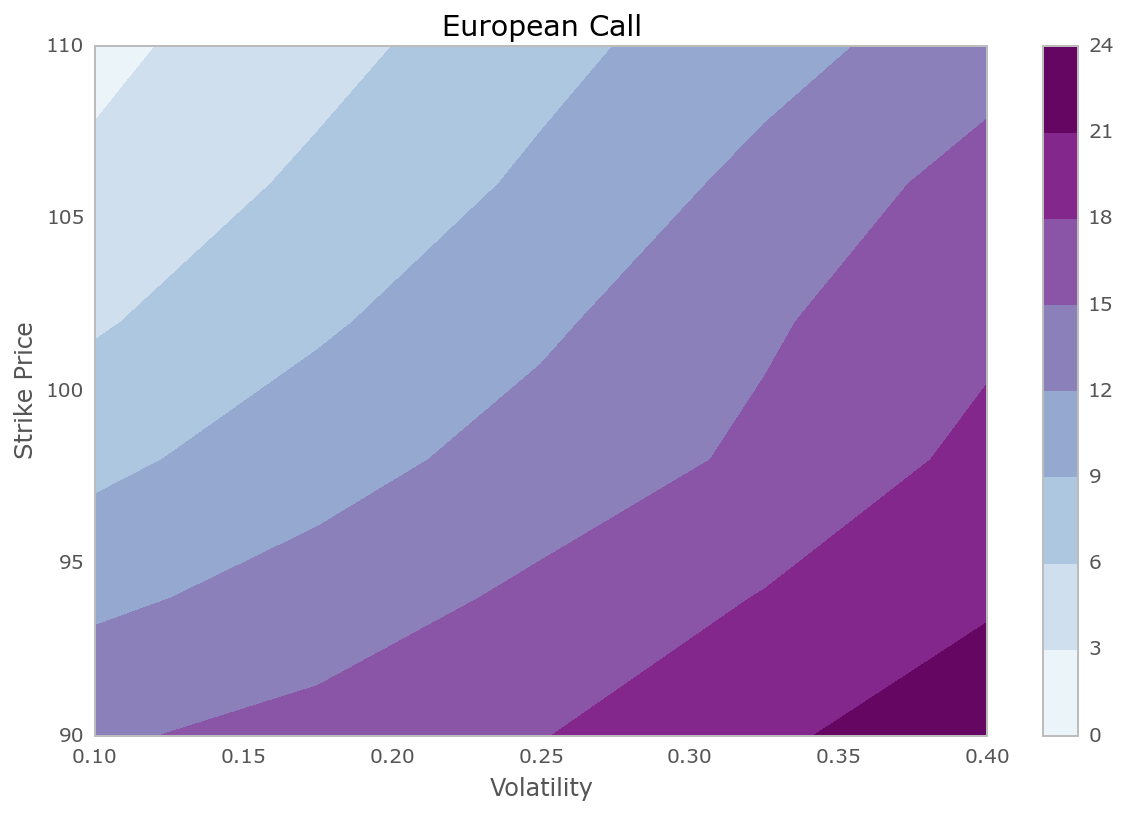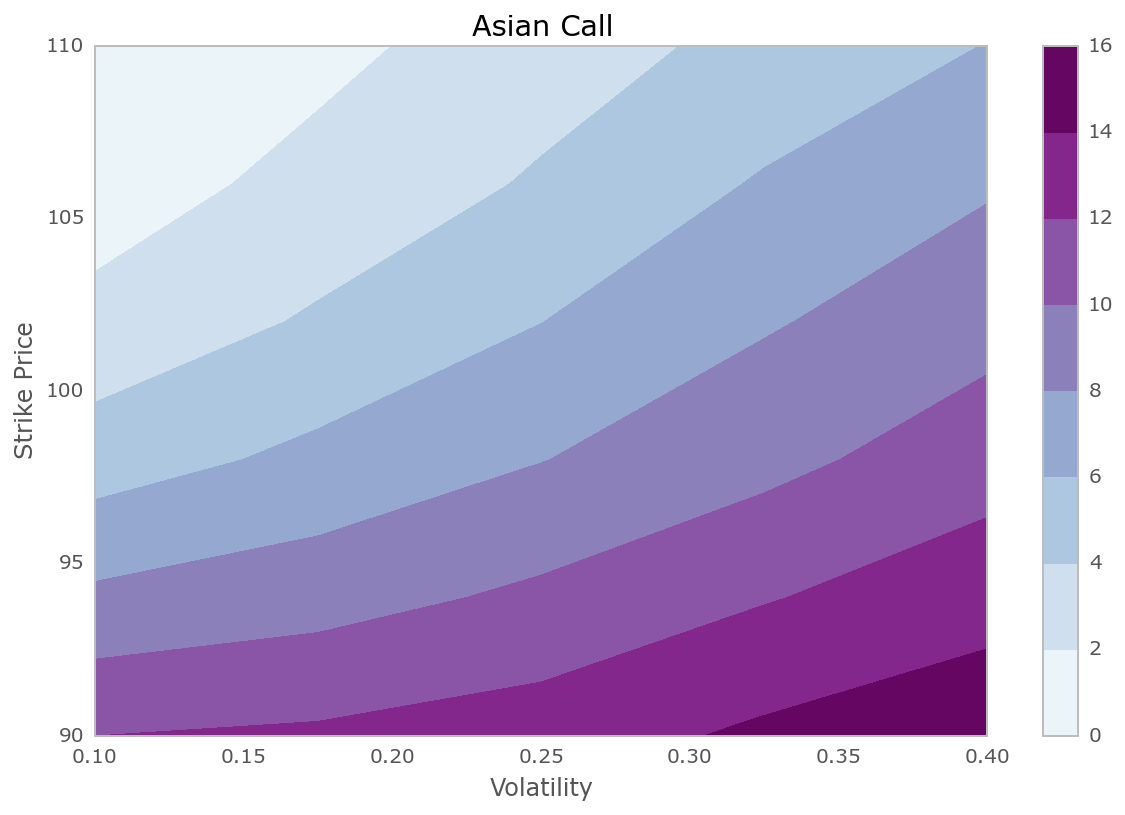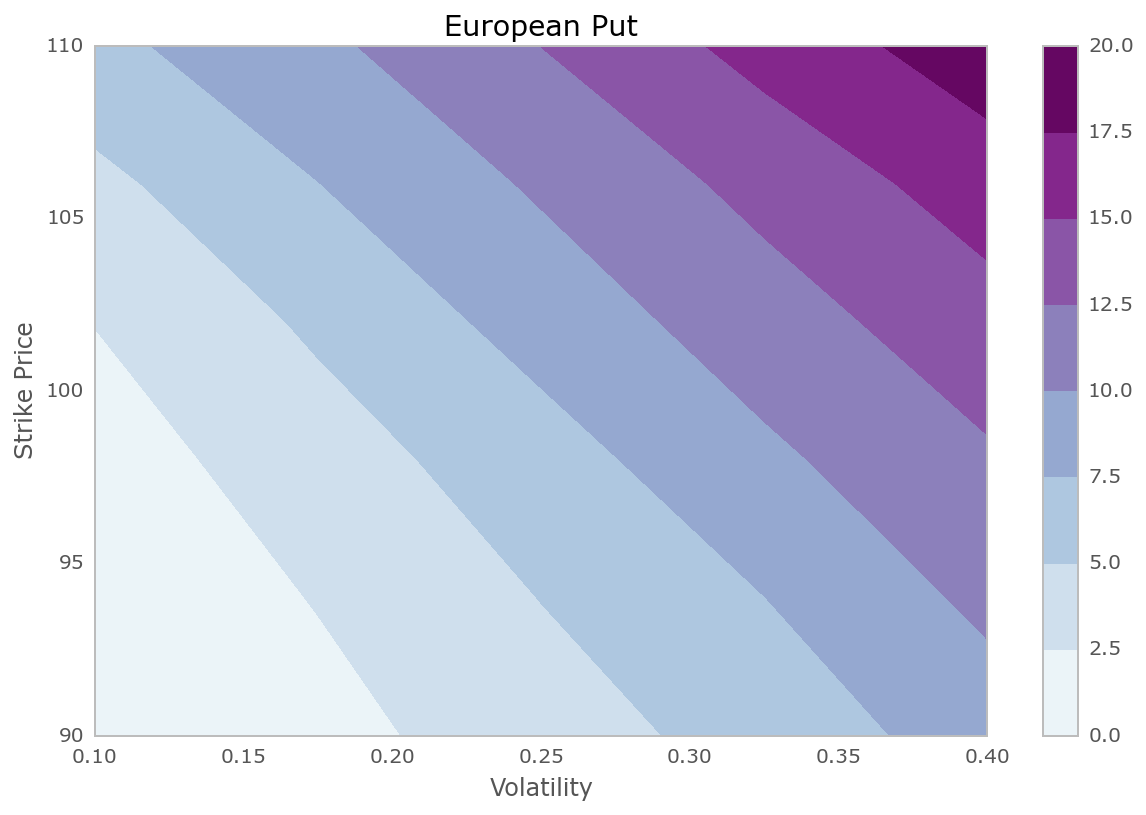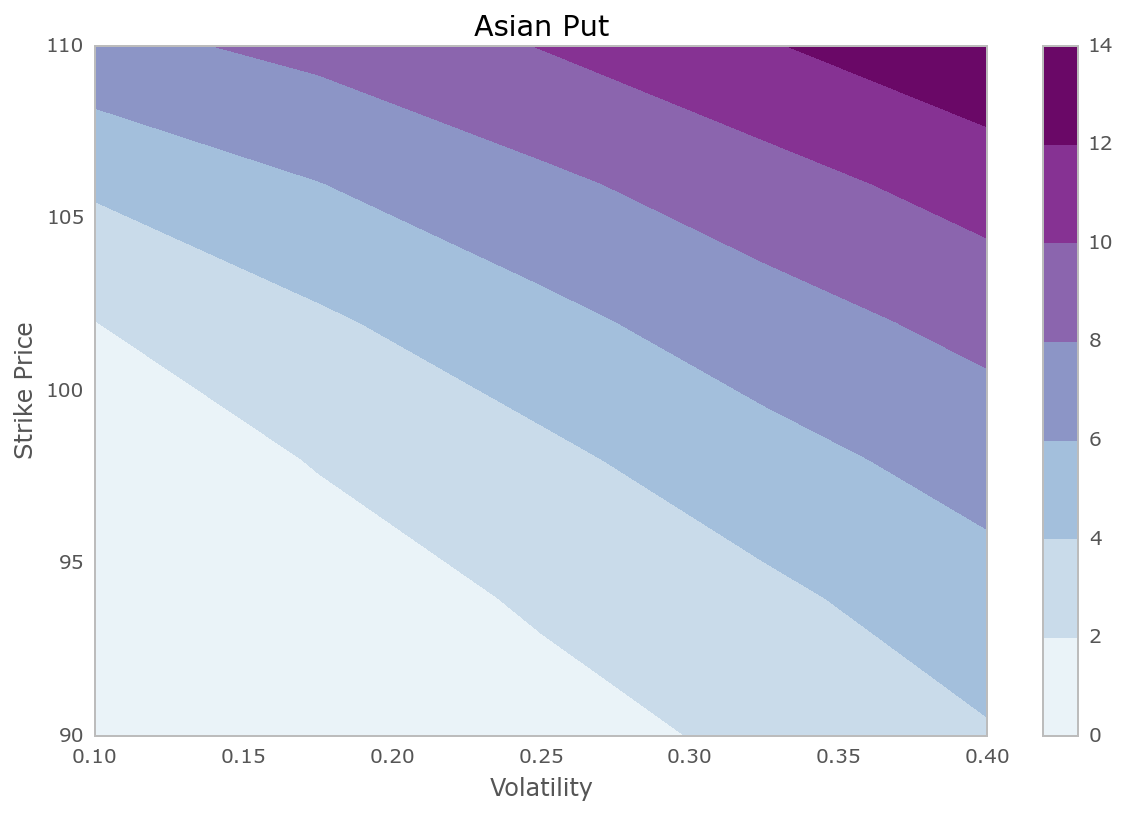# Parallel Monto-Carlo options pricing¶

This notebook shows how to use IPython.parallel to do Monte-Carlo options pricing in parallel. We will compute the price of a large number of options for different strike prices and volatilities.

## Problem setup¶

In :
%matplotlib inline
import matplotlib.pyplot as plt

In :
import sys
import time
from IPython.parallel import Client
import numpy as np


Here are the basic parameters for our computation.

In :
price = 100.0  # Initial price
rate = 0.05  # Interest rate
days = 260  # Days to expiration
paths = 10000  # Number of MC paths
n_strikes = 6  # Number of strike values
min_strike = 90.0  # Min strike price
max_strike = 110.0  # Max strike price
n_sigmas = 5  # Number of volatility values
min_sigma = 0.1  # Min volatility
max_sigma = 0.4  # Max volatility

In :
strike_vals = np.linspace(min_strike, max_strike, n_strikes)
sigma_vals = np.linspace(min_sigma, max_sigma, n_sigmas)

In :
print "Strike prices: ", strike_vals
print "Volatilities: ", sigma_vals

Strike prices:  [  90.   94.   98.  102.  106.  110.]
Volatilities:  [ 0.1    0.175  0.25   0.325  0.4  ]


## Monte-Carlo option pricing function¶

The following function computes the price of a single option. It returns the call and put prices for both European and Asian style options.

In :
def price_option(S=100.0, K=100.0, sigma=0.25, r=0.05, days=260, paths=10000):
"""
Price European and Asian options using a Monte Carlo method.

Parameters
----------
S : float
The initial price of the stock.
K : float
The strike price of the option.
sigma : float
The volatility of the stock.
r : float
The risk free interest rate.
days : int
The number of days until the option expires.
paths : int
The number of Monte Carlo paths used to price the option.

Returns
-------
A tuple of (E. call, E. put, A. call, A. put) option prices.
"""
import numpy as np
from math import exp,sqrt

h = 1.0/days
const1 = exp((r-0.5*sigma**2)*h)
const2 = sigma*sqrt(h)
stock_price = S*np.ones(paths, dtype='float64')
stock_price_sum = np.zeros(paths, dtype='float64')
for j in range(days):
growth_factor = const1*np.exp(const2*np.random.standard_normal(paths))
stock_price = stock_price*growth_factor
stock_price_sum = stock_price_sum + stock_price
stock_price_avg = stock_price_sum/days
zeros = np.zeros(paths, dtype='float64')
r_factor = exp(-r*h*days)
euro_put = r_factor*np.mean(np.maximum(zeros, K-stock_price))
asian_put = r_factor*np.mean(np.maximum(zeros, K-stock_price_avg))
euro_call = r_factor*np.mean(np.maximum(zeros, stock_price-K))
asian_call = r_factor*np.mean(np.maximum(zeros, stock_price_avg-K))
return (euro_call, euro_put, asian_call, asian_put)


We can time a single call of this function using the %timeit magic:

In :
%timeit -n1 -r1 print price_option(S=100.0, K=100.0, sigma=0.25, r=0.05, days=260, paths=10000)

(12.478072469211625, 7.5692079226372924, 6.9498346596114704, 4.5592719279729934)
1 loops, best of 1: 111 ms per loop


## Parallel computation across strike prices and volatilities¶

The Client is used to setup the calculation and works with all engines.

In :
rc = Client()


A LoadBalancedView is an interface to the engines that provides dynamic load balancing at the expense of not knowing which engine will execute the code.

In :
view = rc.load_balanced_view()


Submit tasks for each (strike, sigma) pair. Again, we use the %%timeit magic to time the entire computation.

In :
async_results = []

In :
%%timeit -n1 -r1

for strike in strike_vals:
for sigma in sigma_vals:
# This line submits the tasks for parallel computation.
ar = view.apply_async(price_option, price, strike, sigma, rate, days, paths)
async_results.append(ar)

rc.wait(async_results)  # Wait until all tasks are done.

1 loops, best of 1: 810 ms per loop

In :
len(async_results)

Out:
30

## Process and visualize results¶

Retrieve the results using the get method:

In :
results = [ar.get() for ar in async_results]


Assemble the result into a structured NumPy array.

In :
prices = np.empty(n_strikes*n_sigmas,
dtype=[('ecall',float),('eput',float),('acall',float),('aput',float)]
)

for i, price in enumerate(results):
prices[i] = tuple(price)

prices.shape = (n_strikes, n_sigmas)


Plot the value of the European call in (volatility, strike) space.

In :
plt.figure()
plt.contourf(sigma_vals, strike_vals, prices['ecall'])
plt.axis('tight')
plt.colorbar()
plt.title('European Call')
plt.xlabel("Volatility")
plt.ylabel("Strike Price")

Out:
<matplotlib.text.Text at 0x1100a3290>Plot the value of the Asian call in (volatility, strike) space.

In :
plt.figure()
plt.contourf(sigma_vals, strike_vals, prices['acall'])
plt.axis('tight')
plt.colorbar()
plt.title("Asian Call")
plt.xlabel("Volatility")
plt.ylabel("Strike Price")

Out:
<matplotlib.text.Text at 0x11009b3d0>Plot the value of the European put in (volatility, strike) space.

In :
plt.figure()
plt.contourf(sigma_vals, strike_vals, prices['eput'])
plt.axis('tight')
plt.colorbar()
plt.title("European Put")
plt.xlabel("Volatility")
plt.ylabel("Strike Price")

Out:
<matplotlib.text.Text at 0x1109ef450>Plot the value of the Asian put in (volatility, strike) space.

In :
plt.figure()
plt.contourf(sigma_vals, strike_vals, prices['aput'])
plt.axis('tight')
plt.colorbar()
plt.title("Asian Put")
plt.xlabel("Volatility")
plt.ylabel("Strike Price")

Out:
<matplotlib.text.Text at 0x1109e3b10>